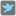• # Pigeons help me please!

## Question related to missionFeed Pigeons

When I [ Run & Check] following code, it's hanged up but Why?

```def checkio(number):
remain = number
pigeons = {}
offset = 0
count = 0

for i in range(number):
offset += count
count += 1
for j in range(count):
pigeons[offset + j] = 0
for k in pigeons:
if remain > 0:
pigeons[k] += 1
remain -= 1

result = 0
for k in pigeons:
if pigeons[k] > 0:
result += 1

return result

#These "asserts" using only for self-checking and not necessary for auto-testing
if __name__ == '__main__':
assert checkio(1) == 1, "1st example"
assert checkio(2) == 1, "2nd example"
assert checkio(5) == 3, "3rd example"
assert checkio(10) == 6, "4th example"
```